Courses

# Notes | EduRev

## NEET : Notes | EduRev

The document Notes | EduRev is a part of the NEET Course Physics 28 Years Past year papers for NEET/AIPMT Class 11.
All you need of NEET at this link: NEET

Q 1. Body A of mass 4m moving with speed u collides with another body B of mass 2m, at rest. The collision is head on and elastic in nature. After the collision the fraction of energy lost by the colliding body A is:    
A: 1/9
B: 8/9
C: 4/9
D: 5/9
Ans:
B
Solution: Fractional loss of KE of colliding body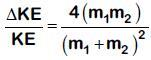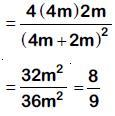Q 2. A disc of radius 2 m and mass 100 kg rolls on a horizontal floor. Its centre of mass has speed of 20 cm/s. How much work is needed to stop it?    
A: 3 J
B: 30 kJ
C: 2 J
D: 1 J
Ans:
A
Solution: Work required = change in kinetic energy Final KE = 0
Initial KE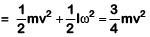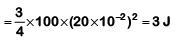|ΔKE| = 3 J

Q 3. A force F = 20 + 10 y acts on a particle in y direction where F is in newton and y in meter. Work done by this force to move the particle from y = 0 to y = 1 m is    
A: 30 J
B: 5 J
C: 25 J
D: 20 J
Ans
: C
Solution: Work done by variable force is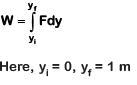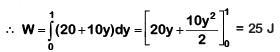Q 4. The work done to raise a mass m from the surface of the earth to a height h, which is equal to the radius of the earth, is:    
A: mgR
B: 2mgR
C: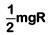D: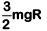Ans:
C
Solution: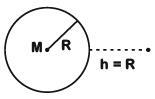Initial potential energy at earths surface is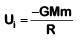Final potential energy at height h = R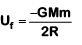As work done = Change in PE∴ W = Uf – Ui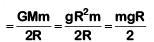(∵ GM = gR2)

Q 5. A moving block having mass m, collides with another stationary block having mass 4m. The lighter block comes to rest after collision. When the initial velocity of the lighter block is v, then the value of coefficient of restitution (e) will be :-    
A: 0.5
B: 0.25
C: 0.8
D: 0.4
Ans
: B
Solution: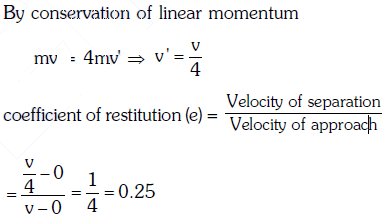Q 6. A body initially at rest and sliding along a frictionless track from a height h (as shown in the figure) just completes a vertical circle of diameter AB = D. The height h is equal to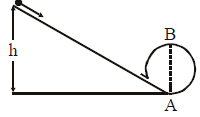A: 3/2 D
B: D
C: 7/5 D
D: 5/4 D
Ans:
D
Solution: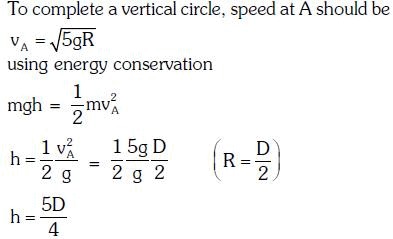Q 7. The kinetic energies of a planet in an elliptical orbit about the Sun, at positions A, B and C are KA, KB and KC respectively. AC is the major axis and SB is perpendicular to AC at the position of the Sun S as shown in the figure. Then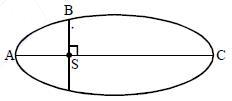A: KA < KB < KC
B: KA > KB > KC
C: KB < KA < KC
D: KB > KA > KC
Ans:
B
Solution: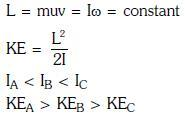Q 8. A spring of force constant k is cut into lengths of ratio 1: 2 : 3. They are connected in series and the new force constant is k'. Then they are connected in parallel and force constant is k''. Then k' : k'' is    
A: 1 : 6
B: 1 : 9
C: 1 : 11
D: 1 : 14
Ans:
C
Solution:
Force constant of spring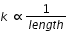after cutting the spring in the ratio 1:2:3, the force constants will be in the ratio 6:3:2
Force constant in series K' is given by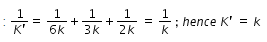Force constant in parallel K" : 6k+3k+2k = 11k;
hence K'/K" = 1/11

Q 9. Consider a drop of rain water having mass 1 g falling from a height of 1 km. It hits the ground with a speed of 50 m/s. Take 'g' constant with a value 10 m/s2. The work done by the    
(i) gravitational force and the
(ii) resistive force of air is :-
A: (i) 1.25 J (ii) – 8.25 J
B: (i) 100 J (ii) 8.75 J
C: (i) 10 J (ii) – 8.75 J
D: (i) – 10 J (ii) – 8.25 J
Ans:
C
Solution: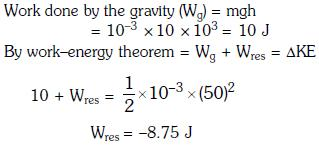Q 10. A body of mass 1 kg begins to move under the action of a time dependent force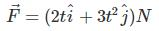, when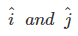are unit vectors along x and y axis. What power will be developed by the force at the time t ?    
A: (2t3+ 3t5)W
B: (2t2 + 3t3)W
C: (2t2 + 4t4)W
D: (2t3 + 3t4)W
Ans:
A
Solution: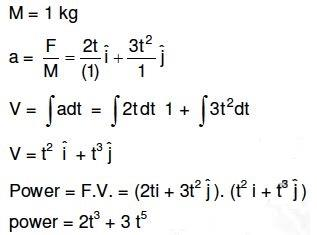Q 11. Two particles of masses m1, m2 move with initial velocities u1 and u2. On collision, one of the particles get excited to higher level, after absorbing energy ε. If final velocities of particles be v1 and v2 then we must have :    
A: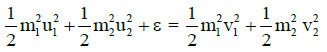B: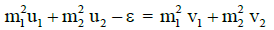C: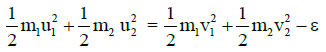D: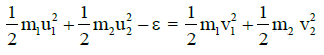Ans:
D
Solution: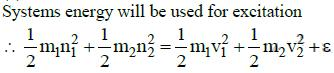Q 12. Two similar springs P and Q have spring constants KP and KQ, such that KP > KQ. They are stretched, first by the same amount (case a), then by the same force (case b). The work done by the springs WP and WQ are related as, in case (a) and case (b), respectively:    
A: WP < WQ; WQ < WP
B: WP = WQ; WP > WQ
C: WP = WQ; WP = WQ
D: WP > WQ; WQ > WP
Ans:
D
Solution: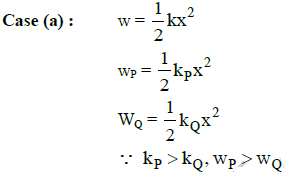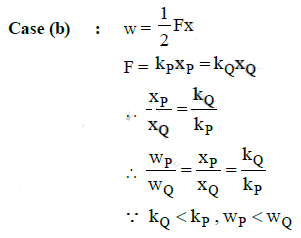Q 13. A mass m moves in a circle on a smooth horizontal plane with velocity v0 at a radius R0. The mass is attached to a string which passes through a smooth hole in the plane as shown.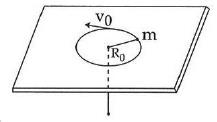The tension in the string is increased gradually and finally m moves in a circle of radius R0/2. The final value of the kinetic energy is :    
A: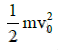B: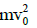C: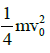D: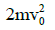Ans:
D
Solution:
When a mass moves in a circle of radius R0 with velocity v0, its kinetic energy is given by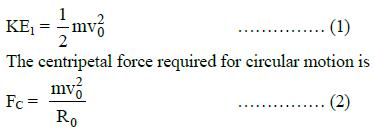The tension in the string is gradually increased and the radius of the circle decreased to R0/2. When the radius of the circle is R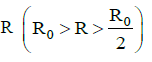the tension in the string is the same as the centripetal force.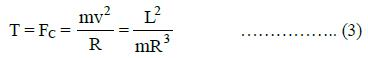where L = mRv is the angular momentum which is conserved.
Work done in reducing the radius of the circle from R0 to R0/2 is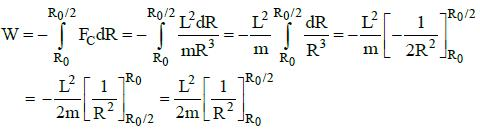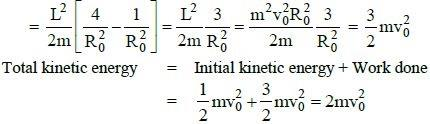Q 14. A block of mass 10 kg, moving in x direction with a constant speed of 10 ms-1, is subjected to a retarding force F = 0.1 x J/m during its travel from x = 20 m to 30 m. Its final KE will be :    
A: 250 J
B: 475 J
C: 450 J
D: 275 J
Ans:
B
Solution:
The block of mass M = 10 kg is moving in the x - direction with a speed v = 10 m/s. Its initial kinetic energy is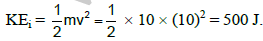It is subjected to a retarding force F = 0.1 x J/m during its travel from x = 20 m to 30 m.
Work done is given by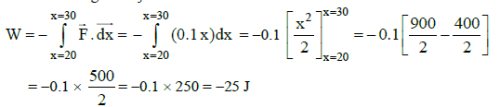Final kinetic energy is, KEf = KEi + W = 500 - 25 = 475

Offer running on EduRev: Apply code STAYHOME200 to get INR 200 off on our premium plan EduRev Infinity!

,

,

,

,

,

,

,

,

,

,

,

,

,

,

,

,

,

,

,

,

,

;